• 2021-08-25 11:39:37

# 一、散点数据插值

使用场景：用站点数据插值成网格数据时，可以使用散点数据插值方法
参考链接：https://ww2.mathworks.cn/help/matlab/ref/scatteredinterpolant.html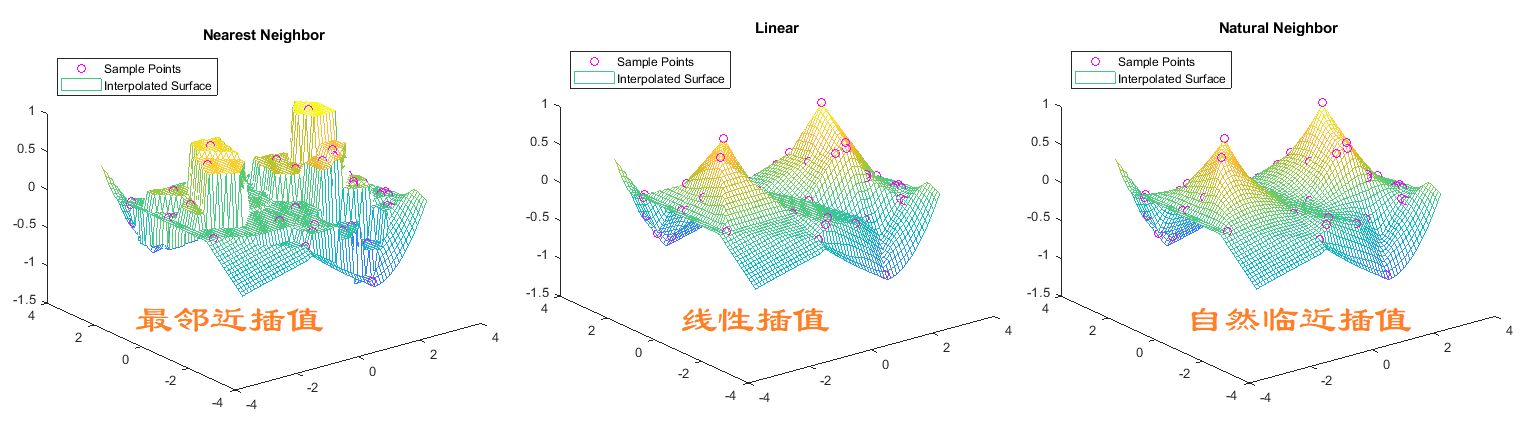## 1.1 简要举例

1. 创建包含 50 个散点的样本数据集。
x = -3 + 6*rand(50,1);
y = -3 + 6*rand(50,1);
v = sin(x).^4 .* cos(y);

2. 创建插值和查询点网格
F = scatteredInterpolant(x,y,v);
[xq,yq] = meshgrid(-3:0.1:3);

3. 绘制结果
F.Method = 'nearest';
vq1 = F(xq,yq);
plot3(x,y,v,'mo')
hold on
mesh(xq,yq,vq1)
title('Nearest Neighbor')
legend('Sample Points','Interpolated Surface','Location','NorthWest')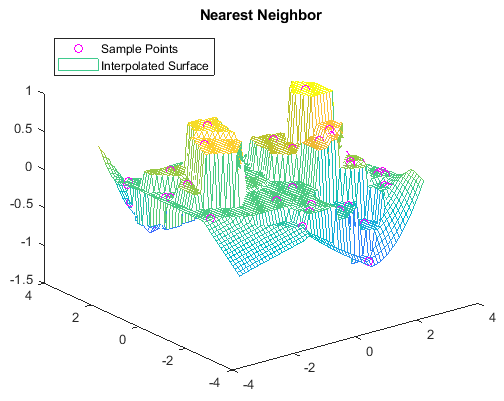## 1.2 可选的插值方法

注意：可以选择插值方法和“外插方法

Method说明连续性
‘linear’（默认值）线性插值C0
‘nearest’最近邻点插值不连续
‘natural’自然邻点插值C1（样本点处除外）
ExtrapolationMethod说明
‘linear’基于边界梯度的线性外插。Method 为 ‘linear’ 或 ‘natural’ 时的默认值。
‘nearest’最近邻点外插。此方法的计算结果为边界处的最近邻点的值。Method 为 ‘nearest’ 时的默认值。
‘none’无外插。Points 凸包之外的任何查询返回 NaN。

# 二、网格数据插值

使用场景：粗分辨率网格降尺度为细分辨率网格时可用
参考链接：https://ww2.mathworks.cn/help/matlab/ref/griddedinterpolant.html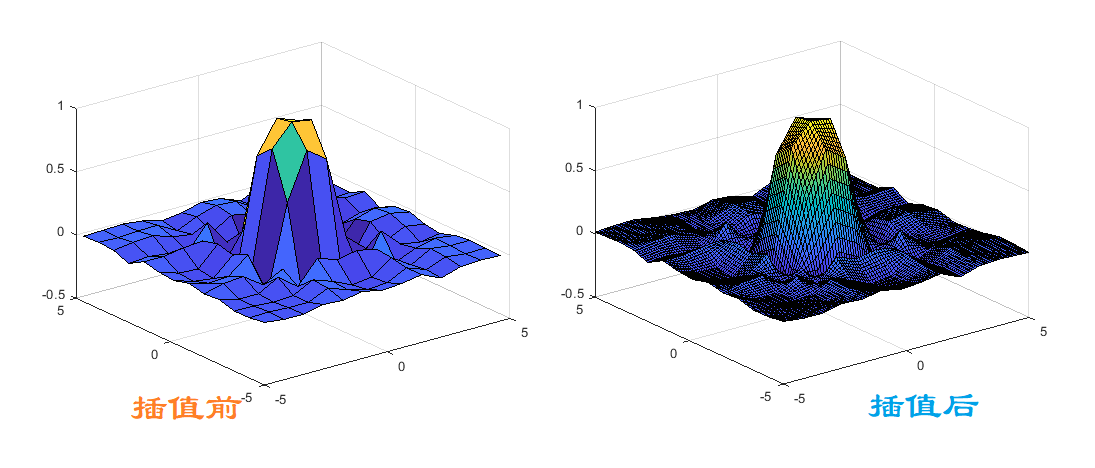## 2.1 简要举例

1. 创建网格样本点
[x,y] = ndgrid(-5:0.8:5);
z = sin(x.^2 + y.^2) ./ (x.^2 + y.^2);
surf(x,y,z)

1. 构建插值结构
F = griddedInterpolant(x,y,z);

1. 插值以提高分辨率
[xq,yq] = ndgrid(-5:0.1:5);
vq = F(xq,yq);
surf(xq,yq,vq)


## 2.2 可选插值方法

MethodValueValueValue
‘linear’（默认值）线性插值。在查询点插入的值基于各维中邻点网格点处数值的线性插值C0每个维需要至少 2 个网格点。比 ‘nearest’ 需要更多内存
‘nearest’最近邻点插值。在查询点插入的值是距样本网格点最近的值。不连续每个维度需要 2 个网格点。内存要求最低，计算速度最快
‘next’下一个邻点插值（仅限于一维）。在查询点插入的值是下一个抽样网格点的值。不连续需要至少 2 个点。内存要求和计算时间与 ‘nearest’ 相同
‘previous’上一个邻点插值（仅限于一维）。在查询点插入的值是上一个抽样网格点的值。不连续需要至少 2 个点。内存要求和计算时间与 ‘nearest’ 相同
‘pchip’保形分段三次插值（仅限一维）。在查询点插入的值基于邻点网格点处数值的保形分段三次插值。C1需要至少 4 个点。比 ‘linear’ 需要更多内存和计算时间
‘cubic’三次插值。在查询点插入的值基于各维中邻点网格点处数值的三次插值。插值基于三次卷积。C1网格必须有均匀间距，虽然每个维度上的间距不必相同。每维需要至少 4 个网格点。比 ‘linear’ 需要更多内存和计算时间
‘makima’修正 Akima 三次 Hermite 插值。在查询点插入的值基于次数最大为 3 的多项式的分段函数，使用各维中相邻网格点的值进行计算。为防过冲，已修正 Akima 公式。C1每一维需要至少 2 个点。产生的波动比 ‘spline’ 小，但不像 ‘pchip’ 那样急剧变平。计算成本高于 ‘pchip’，但通常低于 ‘spline’。内存要求与 ‘spline’ 类似
‘spline’三次样条插值。在查询点插入的值基于各维中邻点网格点处数值的三次插值。插值基于使用非结终止条件的三次样条。C2每维需要 4 个网格点。比 ‘cubic’ 需要更多内存和计算时间

ExtrapolationMethod - 外插方法
‘linear’ （默认） | ‘nearest’ | ‘next’ | ‘previous’ | ‘pchip’ | ‘cubic’ | ‘spline’ | ‘makima’ | ‘none’
外插方法，指定为 ‘linear’、‘nearest’、‘next’、‘previous’、‘pchip’、‘cubic’、‘spline’ 或 ‘makima’。另外，如果您希望网格域之外的查询返回 NaN 值，可以指定 ‘none’。

如果您省略 ExtrapolationMethod，则默认值是为 Method 指定的值。如果您同时省略 Method 和 ExtrapolationMethod 参数，则两个值均默认为 ‘linear’

# 总结及参考

（1）注意：可以选择插值方法和“外插方法
（2） 如果存在大量样本点或查询点，或者担心内存使用量太大，您可以使用网格向量来提高内存使用率。

与interp2/3的差别
interp2要求矩形网格（meshgrid）；
griddata的插值网格可以随意，包括矩形网格和非矩形网格；
griddedInterpolant 比 interp2 更高效一些；更多相关内容
• 该文件含有MATLAB代码的样条插值，三次样条插值，以及线性插值，并可以通过运行进行比较
• 利用matlab插值法实现序列信号的包络，y为信号序列，添加之后可以直接运行成功。给那些需要的人仅供参考，如有异议，可以交流。
• ## MATLAB插值

千次阅读 2021-04-18 04:43:24
在信号处理和图形分析中，插值运算的应用较为广泛，MATLAB提供了多种插值函数，可以满足不同的需求。4.5.1 一维数据插值一维数据插值常使用函数interp1，其一般的语法格式为：yi=interp1(x,y,xi,method)。其中y为函...

4.5  插值

插值就是在已知数据之间计算估计值的过程，是一种实用的数值方法，是函数逼近的重要方法。在信号处理和图形分析中，插值运算的应用较为广泛，MATLAB提供了多种插值函数，可以满足不同的需求。

4.5.1  一维数据插值

一维数据插值常使用函数interp1，其一般的语法格式为：yi=interp1(x,y,xi,method)。其中y为函数值矢量，x为自变量的取值范围，x与y的长度必须相同；xi为插值点的向量或者数组，method为插值方法选项。对于插值，MATLAB提供了如下几种方法。

(1)邻近点插值(method=’nearest’)。

(2)线性插值(method=’linear’)：在两个数据点之间连接直线，计算给定的插值点在直线上的值作为插值结果，该方法是interp1函数的默认方法。

(3)三次样条插值(method=’spline’)：通过数据点拟合出三次样条曲线，计算给定的插值点在曲线上的值作为插值结果。

(4)立方插值(method=’pchip’or’cubic’)：通过分段立方Hermite插值方法计算插值结果。

选择一种插值方法时，考虑的因素包括运算时间、占用计算机内存和插值的光滑程度。一般来说：

(5)邻近点插值方法的速度最快，但平滑性最差；

(6)线性插值方法占用的内存较邻近点插值方法多，运算时间也稍长，与邻近点插值不同，其结果是连续的，但顶点处的斜率会改变；

(7)三次样条插值方法的运算时间最长，但内存的占用较立方插值法要少，但其插值数据和导数都是连续的。在这4种方法中，三次样条插值结果的平滑性最好，但如果输入数据不一致或数据点过近，就可能出现很差的插值效果。

【例4-39】  一维插值函数interp1应用与比较示例。

>> x=0:10;

>> y=cos(x);

>> xi=0:0.25:10;

>> strmod={'nearest','linear','spline',' pchip'}%  将插值方法存储到元胞数组

'nearest'    'linear'    'spline'   'pchip'

>> strlb={'(a)method=nearest','(b)method=linear',...

'(c)method=spline','(d)method=pchip'}              %  绘图标签

Columns 1through 2

'(a)method=nearest'   '(b)method=linear'

Columns 3through 4

'(c)method=spline'    '(d)method=pchip'

>> for i=1:4

yi=interp1(x,y,xi,strmod{i});                     %  插值

subplot(2,2,i)                                        %  子图

plot(x,y,'ro',xi,yi,'b'),xlabel(strlb(i))         %  绘图

本例创建了元胞数组strmod来存储4种用到的插值方法{'nearest','linear','spline','pchip'}，然后通过循环来调用插值函数interp1，最终插值的结果用图形来对比。一维插值结果比较如图4-4所示。可以看出，三次样条插值结果的平滑性最好，而邻近点插值效果最差。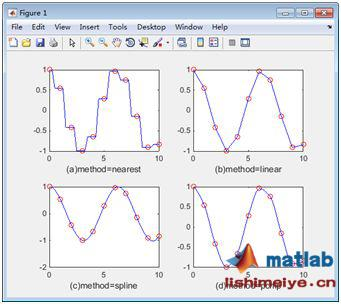图4-4  一维插值方法结果比较

4.5.2  二维数据插值

二维插值也是常用的插值运算方法，主要应用于图形图像处理和三维曲线拟合等领域。二维插值由函数interp2实现，其一般语法为：zi=interp2(x,y,z,xi,yi,method)。

其中x和y为由自变量组成的数组，x与y的尺寸相同，z为二者相对应的函数值；xi和yi为插值点数组，method为插值方法选项。interp1函数中的4种插值方法也可以在interp2函数中使用。

【例4-40】  二维插值函数interp2应用与比较示例。

[x,y,z]=peaks(6);                                  %  MATLAB自带的测试函数

mesh(x,y,z)                                        %  绘制原始数据图

title('原始数据')

[xi,yi]=meshgrid(-3:0.2:3,-3:0.2:3);               %  生成供插值的数据网格

strmod={'nearest','linear','spline','cubic'};     %  将插值方法存储到元胞数组

strlb={'(a)method=nearest','(b)method=linear',...

'(c)method=spline','(d)method=cubic'};       %  绘图标签

figure                                           % 建立新绘图窗口

zi=interp2(x,y,z,xi,yi,strmod{i});       % 插值

subplot(2,2,i)

mesh(xi,yi,zi);                             %  绘图

title(strlb{i})                              %  图标题

本例计算了调用'nearest'、'linear'、'spline'和'cubic'等4种方法进行插值，其中原始数据如图4-5所示，插值之后的结果如图4-6所示。由结果图可以看出，各种插值方法的精度是不同的。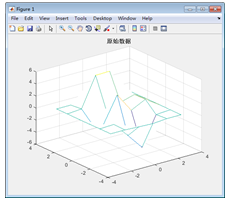图4-5 二维插值原始数据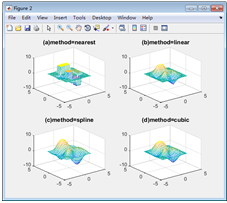图4-6  二维插值结果

4.5.3  多维插值

多维插值包括三维插值函数interp3和n维插值函数interpn，其函数的调用方式及插值方法与一维、二维插值基本相同。这里以三维为例，其一般格式为：

zi=interp3(x,y,z,v,xi,yi,zi,method)

其中x、y、z为由自变量组成的数组，x、y、z的尺寸相同，v为相应的函数值；xi、yi、zi为插值点数组，method为插值方法选项。和一维插值的4种方法一致。

【例4-41】  三维插值函数interp3示例。

>> [x,y,z,v]=flow(8);                             %  flow是MATLAB自带的测试函数

>> slice(x,y,z,v,[3,5],2,[-2,3])                  % 画切片图

>> title('插值前')

>>[xi,yi,zi]=meshgrid(0.1:0.25:10,-3:0.25:3,-3:0.25:3);  %  创建插值点数据网格

>> vi=interp3(x,y,z,v,xi,yi,zi);                            %  插值

>> figure

>> slice(xi,yi,zi,vi,[3,5],2,[-2,3])                        %  画插值后切片图

>> title('插值后')

插值前的flow函数如图4-7所示，进行三维插值之后的结果如图4-8所示。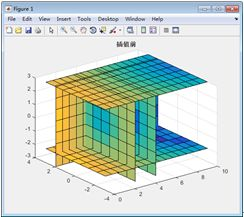图4-7 插值前函数图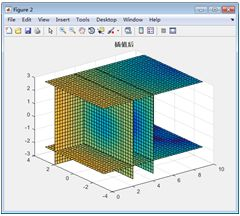图4-8  插值后函数图

4.5.4  样条插值

样条函数产生的基本思想是：设有一组已知的数据点，目标是找一组拟合多项式。在拟合过程中，对于此数据组的每个相邻样点对(Breakpoints)，用三次多项式去拟合样点之间的曲线。为保证拟合的唯一性，对该三次多项式在样点处的一阶、二阶导数加以约束。这样除被研究区间端点外，所有内样点处可保证样条有连续的一阶、二阶导数。

MATLAB中提供了spline函数来进行样条插值。spline函数的调用语法如下。

(1)yy = spline(x,y,xx)：根据样点数据(x,y)，求xx所对应的三次样条插值。

(2)pp = spline(x,y)：从样点数据(x,y)获得逐段多项式样条函数数据pp。

【例4-42】  样条插值spline函数应用示例。

>> x = -4:4;

>> y = [0 .15 1.12 2.36 2.36 1.46 .49 .060];         %  插值前数据

>> cs = spline(x,[0 y 0]);                              %  插值

>> xx = linspace(-4,4,101);                              % 创建绘图自变量数组

>> plot(x,y,'o',xx,ppval(cs,xx),'-');                  %  绘制结果图

得到的结果如图4-9所示。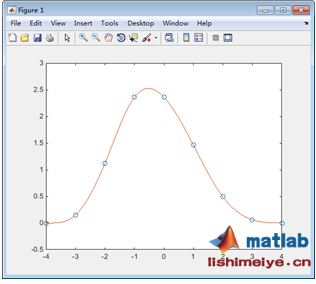图4-9  样条插值

编辑：青樱、蓬荜生辉、倾国倾城

复核：数韵校园工作室如你对话题感兴趣，

展开全文• 学会用一维插值函数yi=interp1(xo,yo,x,’menthod’)求出函数在插值点处的函数值，和用二维函数plot()作图。用二维插值函数zi=interp2(x0,y0,z0,x,y,’method’)求其在网格节点数据的插值，和用三维函数surfc()作图.
• matlab非常方便用于数值计算，本文档详细介绍了matlab用于插值的函数和用法，非常有用。
• matlab有关插值与拟合与绘图命令，有众多代码，有文档，有PPT，可供学习与参考。matlab 插值与拟合与绘图命令
• ## Matlab插值

千次阅读 2020-07-07 14:57:08
插值

1.一维插值函数
matlab中有现成的一维插值函数interp1
语法为

y=interp1(x0,y0,x,'method')


其中method指定插值的方法，默认为线性插值。其值可为

插值方法解释
nearest最近项插值
linear线性插值
spline立方样条插值
cubic立方插值

所有的插值方法要求x0是单调的。

2.三次样条插值
在matlab中数据点称为断点。如果三次样条插值没有边界条件，最常用的方法就是采用非扭结(not-a-knot)条件
matlab中三次样条插值有如下函数

y=interp1(x0,y0,x,'spline');
y=spline(x0,y0,x);
y=csape(x0,y0,conds);
y=csape(x0,y0,conds,valconds);y=fnval(pp,x);


其中：x0，y0是已知数据点；x是插值点；y是插值点的函数值。

对于三次样条插值，提倡使用函数csage。csage的返回值是pp形式，要求插值点的函数值必须调用函数fnval。

pp=esape(x0,y0);%


式中：使用默认的边界条件，即Lagrange边界条件

pp=esape(x0,y0,conds,valconds);


式中：conds指定插值的边界条件，其值可为

conds解释
complete边界为一阶导数，一阶导数的值在valconds参数中给出，若忽略valconds参数，则按照默认情况处理
not-a-knot非扭结条件
periodic周期条件
second边界为二阶导数，二阶导数的值在valconds参数中给出，若忽略valconds参数，二阶导数的默认值为[0,0]
variational设置边界的二阶导数为[0,0]

对于一些特殊的边界条件，可以通过conds的一个1*2的矩阵来表示，conds元素的取值为0、1、2.

插值举例

结果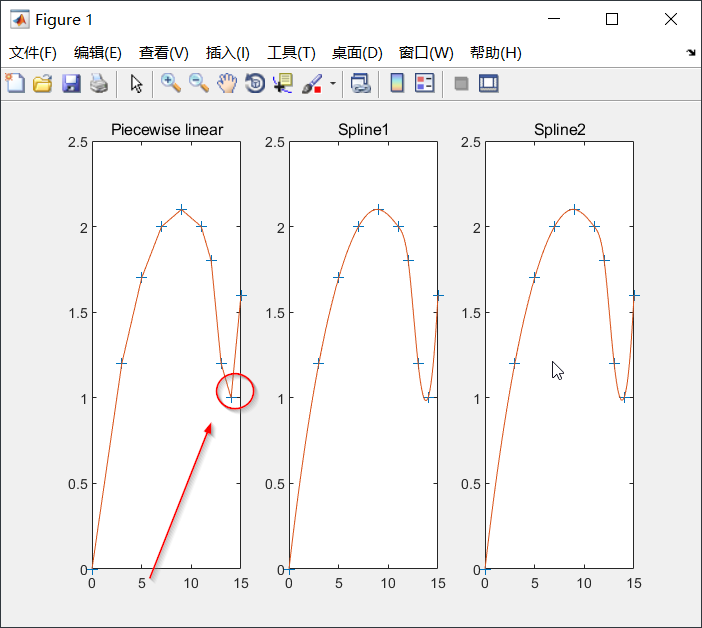结果分析：分段线性插值的光滑性较差（图1），建议选用三次样条插值。

matlab代码

clc;clear;
x0=[0 3 5 7 9 11 12 13 14 15];
y0=[0,1.2 1.7 2.0 2.1 2.0 1.8 1.2 1.0 1.6];

x=0:0.1:15;
y1=interp1(x0,y0,x);%一维插值，默认线性插值

y2=interp1(x0,y0,x,'spline');%立方样条插值

pp1=csape(x0,y0);%三次样条插值，默认使用Lagrange边界条件
y3=fnval(pp1,x);

pp2=csape(x0,y0,'second');%三次样条插值，边界为2阶导数
y4=fnval(pp2,x);
[x',y1',y2',y3',y4']%输出结果

%画图
subplot(1,3,1)
plot(x0,y0,'+',x,y1);
title("Piecewise linear");

subplot(1,3,2)
plot(x0,y0,'+',x,y2);
title("Spline1");

subplot(1,3,3)
plot(x0,y0,'+',x,y3);
title("Spline2");



3.二维插值
若节点是二维，插值函数就是二元函数，即曲面。
1）插值节点为网格节点
已知m*n个节点： ( x i , y j , z i j ) (x_i,y_j,z_{ij}) 求点(x,y)处的插值z。
matlab中有一些计算二维插值的命令

z=interp2(x0,y0,z0,x,y,'method');


其中：x0，y0分别为m维和n维向量，表示节点。z0为n*m矩阵，表示节点值；x，y为一维数组，表示插值点，x与y应是方向不同的向量，即一个是行向量，另一个是列向量；z为矩阵，它的行数为y的维数，列数为x的维数，表示得到的插值；

如果是三次样条插值，可以使用

pp=csape({x0,y0},z0,conds,valconds);
z=fnval(pp,{x,y});


其中： x0,y0 分别为m 维和n维向量，z0 为m × n 维矩阵，z 为矩阵，它的行数为x的维
数，列数为y 的维数，表示得到的插值，具体使用方法同一维插值。
举例：在一丘陵地带测量高程，x 和y 方向每隔100米测一个点，得高程如2表，试插
值一曲面，确定合适的模型，并由此找出最高点和该点的高程。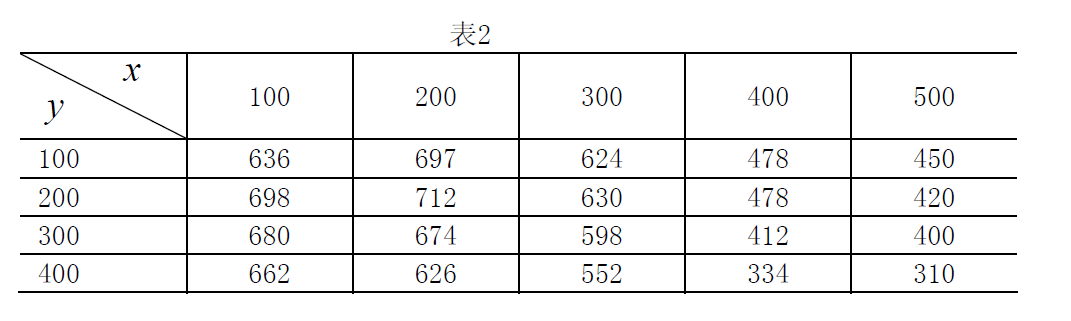clear,clc
x=100:100:500;
y=100:100:400;
z=[636    697    624    478   450
698    712    630    478   420
680    674    598    412   400
662    626    552    334   310];
pp=csape({x,y},z')
xi=100:10:500;yi=100:10:400;
cz=fnval(pp,{xi,yi});
[i,j]=find(cz==max(max(cz)))  %找最高点的地址
x=xi(i),y=yi(j),zmax=cz(i,j)  %求最高点的坐标



运行结果

i =

8

j =

9

x =

170

y =

180

zmax =

720.6252


2）插值节点为散乱节点
已知n个节点 ( x i , y i , z i ) (x_i,y_i,z_i) ,求点(x,y)处的插值z
Matlab 中提供了插值函数griddata

ZI=griddata(x,y,z,XI,YI);


其中x,y,z 均为n 维向量，指明所给数据点的横坐标、纵坐标和竖坐标。向量XI、YI 是给定的网格点的横坐标和纵坐标，返回值ZI 为网格（XI，YI）处的函数值。XI与YI 应是方向不同的向量，即一个是行向量，另一个是列向量。

绘制海底曲面的形状
海底水深数据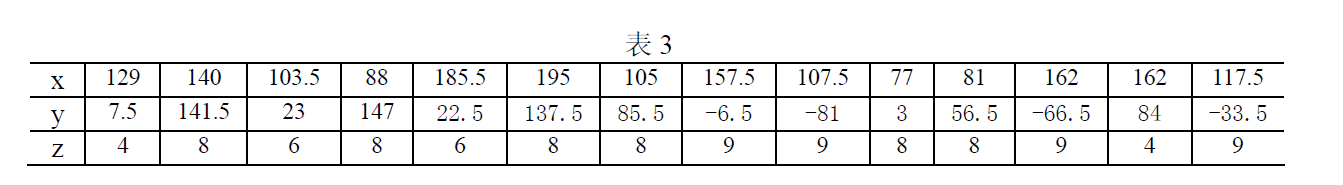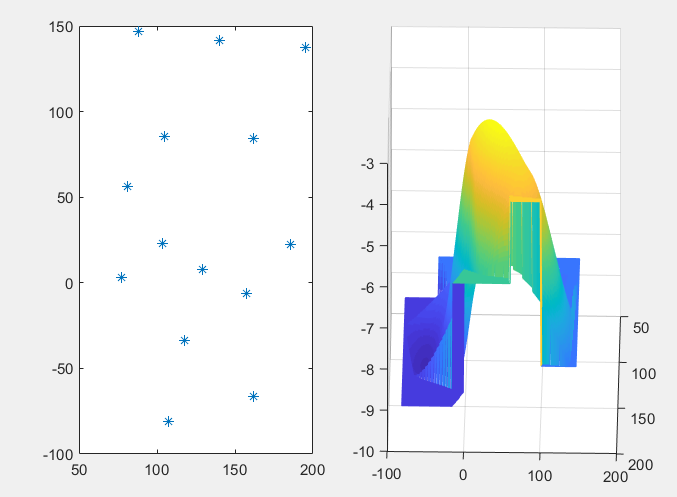clc, clear
x=[129,140,103.5,88,185.5,195,105,157.5,107.5,77,81,162,162,117.5];
y=[7.5,141.5,23,147,22.5,137.5,85.5,-6.5,-81,3,56.5,-66.5,84,-33.5];
z=-[4,8,6,8,6,8,8,9,9,8,8,9,4,9];
xmm=minmax(x)  %求x的最小值和最大值
ymm=minmax(y)  %求y的最小值和最大值
xi=xmm(1):xmm(2);
yi=ymm(1):ymm(2);
zi1=griddata(x,y,z,xi,yi','cubic'); %立方插值
zi2=griddata(x,y,z,xi,yi','nearest'); %最近点插值
zi=zi1;  %立方插值和最近点插值的混合插值的初始值
zi(isnan(zi1))=zi2(isnan(zi1));  %把立方插值中的不确定值换成最近点插值的结果
subplot(1,2,1), plot(x,y,'*')
subplot(1,2,2), mesh(xi,yi,zi)



运行结果

xmm =

77   195

ymm =

-81   147

展开全文• 使用bezier，三次样条，拉格朗日等方法进行插值
• 这是一份关于插值法的ppt，之前学习是用到的资源，现在贡献给大家参考。
• 利用matlab软件进行函数插值的代码，主要利用了interp1
• ## matlab插值拟合

千次阅读 2022-04-30 11:53:42
拟合 插值

# 拟合

运用matlab提供的工具箱，在APP的CurveFitting里面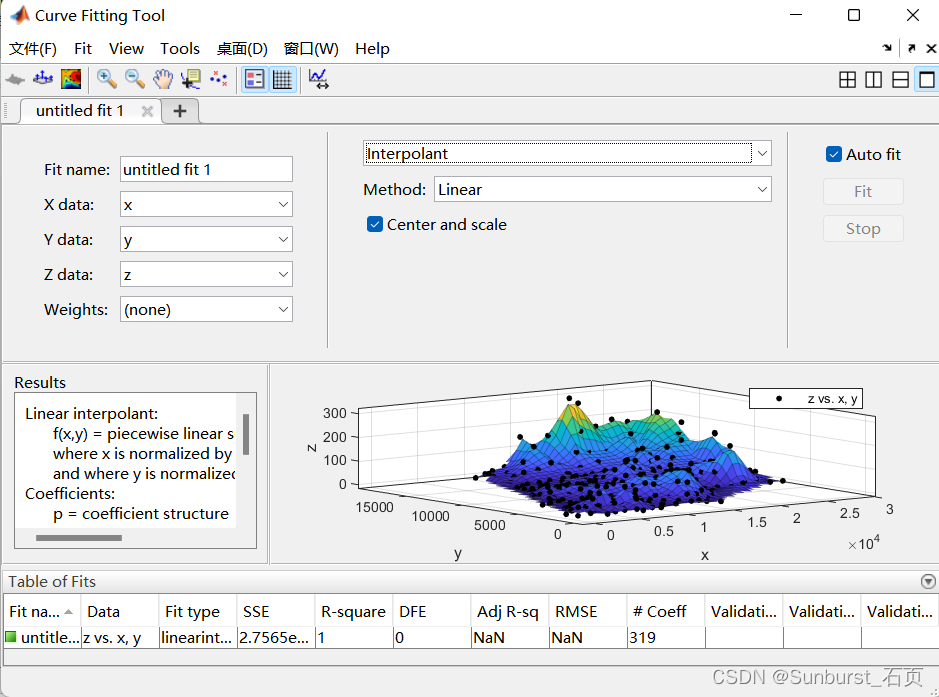# 插值

## 一维插值

### 函数介绍

在这里我们介绍interp1函数的用法：
yi=interp1（x，y，xi，‘method’）
x：已知的自变量的值，是一个向量；
y：与x对应的因变量的值；
xi：用户另外指定的插值点的横坐标
yi：指定的插值点的纵坐标
method：插值方法，这里以三次样条插值为例

### 举例

我们看一个例题：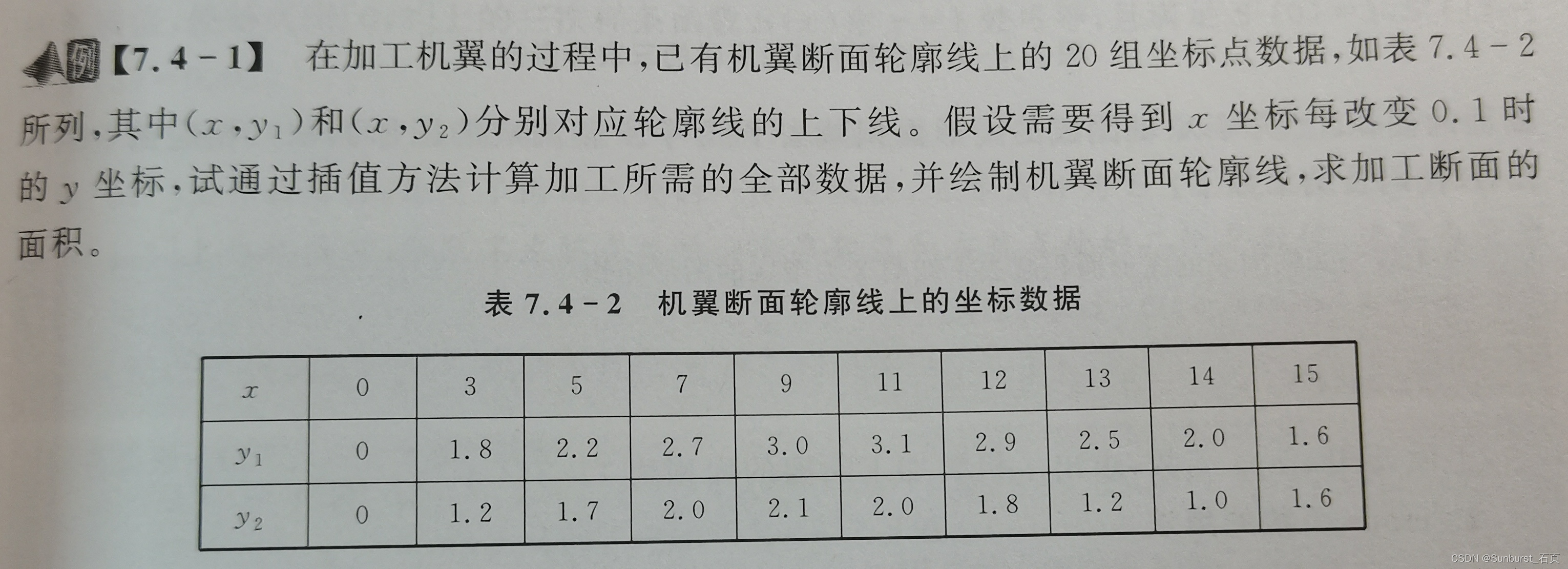解答：机翼断面轮廓是封闭曲线，我们对其上下线进行三次样条插值，matlab代码如下：

>> x0=[0,3,5,7,9,11,12,13,14,15];%已知的x的坐标构成的向量
>> y01=[0,1.8,2.2,2.7,3.0,3.1,2.9,2.5,2.0,1.6];%已知的y坐标上线
>> y02=[0,1.2,1.7,2.0,2.1,2.0,1.8,1.2,1.0,1.6];%已知的y坐标下线
>> x=0:0.1:15;%插值点x的坐标，每间隔0.1插一个值
>> ysp1=interp1(x0,y01,x,'spline');%spline表示三次样条插值方式
>> ysp2=interp1(x0,y02,x,'spline');
>> plot([x0,x0],[y01,y02],'o');%对于每个坐标点标一个圈
>> hold on;%等一下
>> plot(x,ysp1,'r',x,ysp2,'r');%画两条线
>> xlabel('X');%指明横坐标名称
>> ylabel('Y');%指明纵坐标名称
>> legend('插值节点','三次样条插值','location','northwest')%图例然后再用上线减下线即可，matlab提供专门的函数

>> S=trapz(x,ysp1)-trapz(x,ysp2)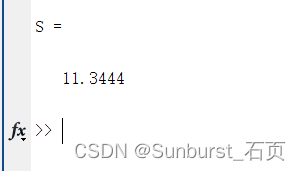## 二维插值

### 网格插值

#### 函数介绍

zi=interp2(x,y,z,xi,yi,‘method’)
z=meshgrid（x，y）：用于生成二维表格

#### 举例

看具体的例子吧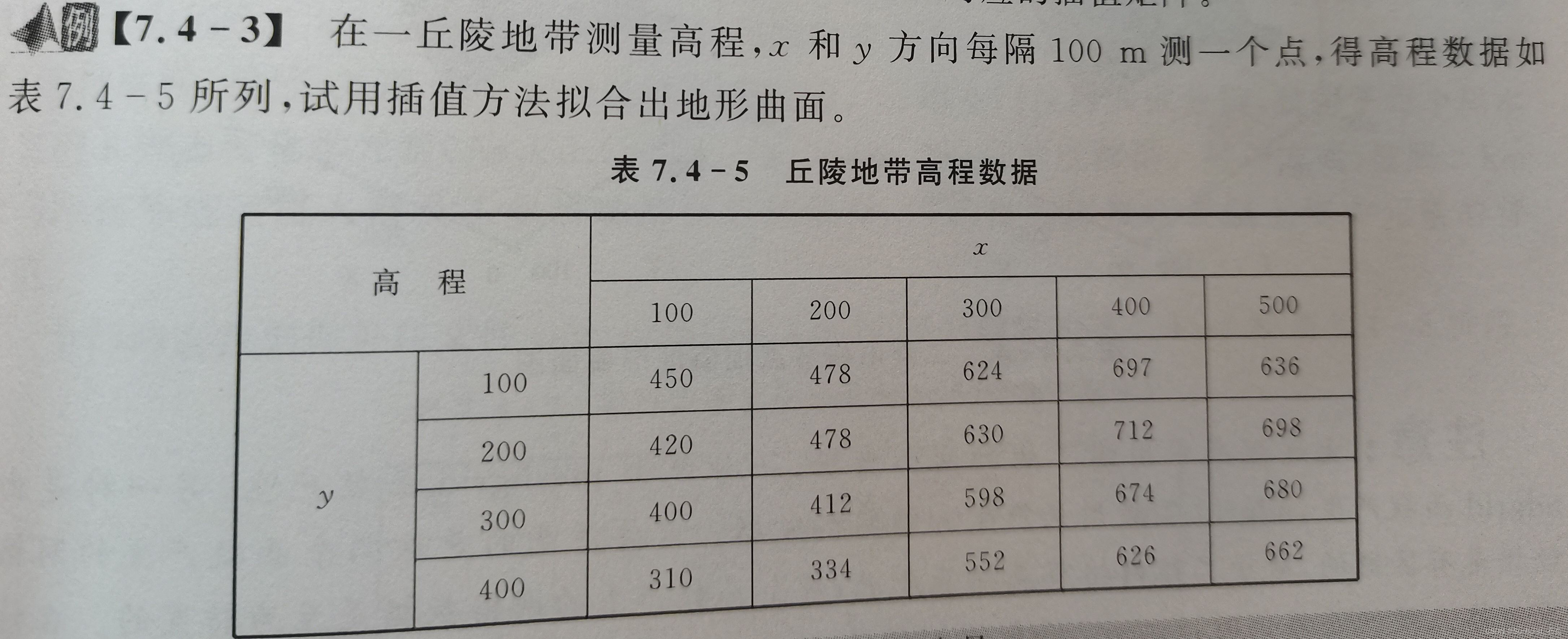代码如下：

x = 100:100:500;
y = 100:100:400;
[X,Y] = meshgrid(x,y);%生成二维网格
Z = [450  478  624  697  636
420  478  630  712  698
400  412  598  674  680
310  334  552  626  662];
xd = 100:20:500;
yd = 100:20:400;
[Xd,Yd] = meshgrid(xd,yd);
figure;
Zd = interp2(X,Y,Z,Xd,Yd,'spline');%进行二维的三次样条插值
surf(Xd,Yd,Zd);%绘图
xlabel('X'); ylabel('Y'); zlabel('Z'); title('interp2')%指明三个坐标轴名称及标题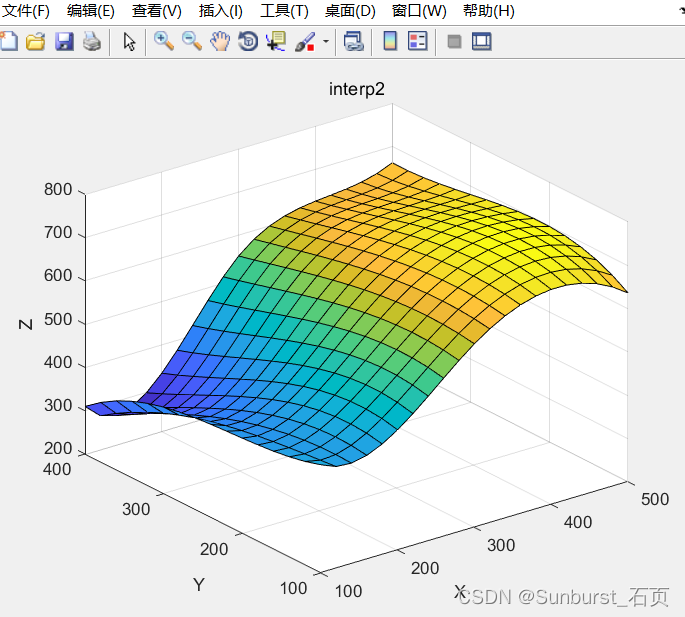### 散点插值

#### 函数介绍

griddata
Zd = griddata(x,y,z,Xd,Yd);x,y和z是已知值，Xd，Yd是要插入的数据，Zd是得到的插值
x=linspace(x1,x2,n):x1是起始值，x2是结束值，n为元素的个数

#### 举例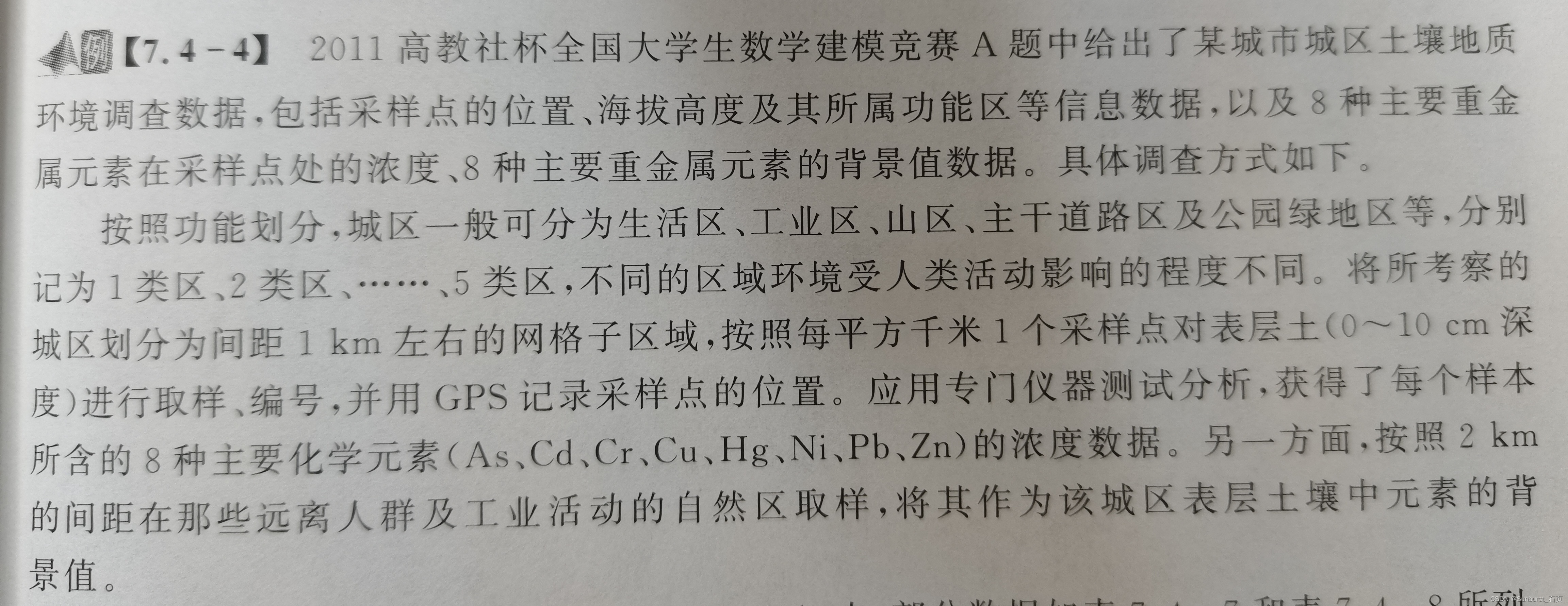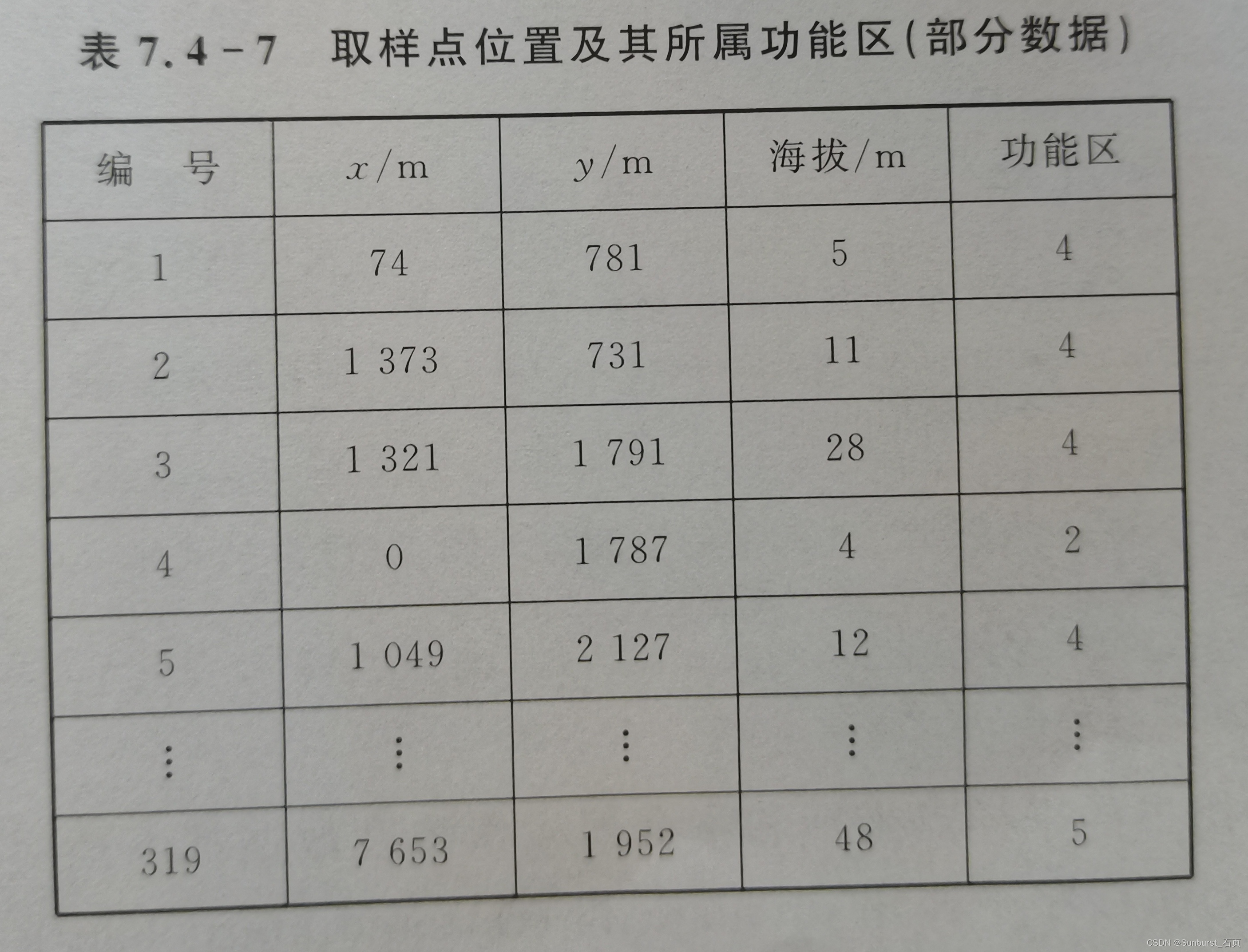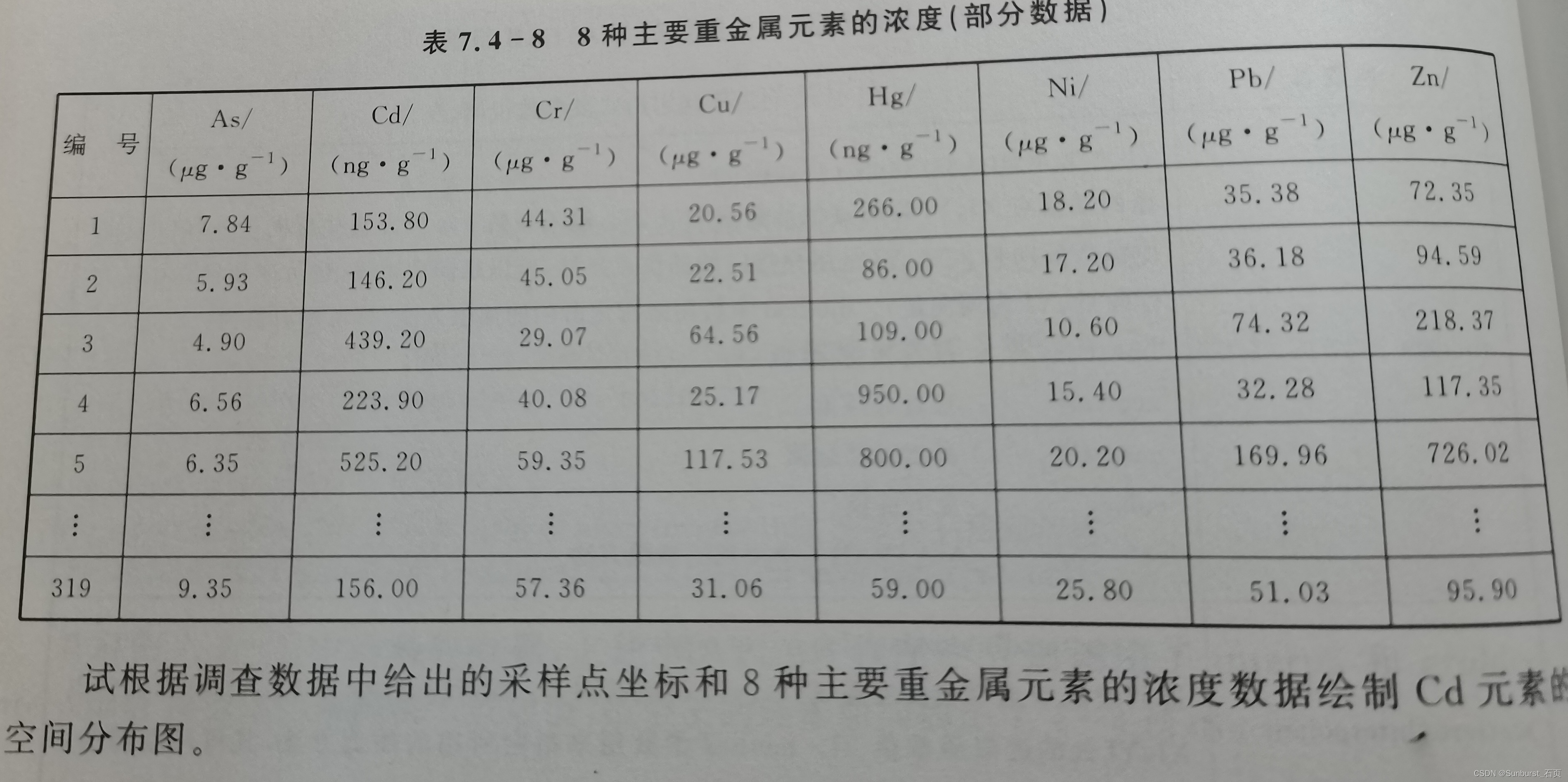过程：
1 计算出插值点上的海拔高度和Cd元素浓度
2 绘制四维图，重金属浓度为第四维
代码如下：

xyz = xlsread('cumcm2011A.xls',1,'B4:D322');%读取sheet1的数据
x = xyz(:,1);%取出第一列的数据，即x
y = xyz(:,2);%取出第二列的数据，即y
z = xyz(:,3);%取出第三列的数据，即海拔
xd = linspace(min(x),max(x),60);
yd = linspace(min(y),max(y),60);
[Xd,Yd] = meshgrid(xd,yd);%生成网格矩阵
Zd = griddata(x,y,z,Xd,Yd);
Cd = griddata(x,y,Cd,Xd,Yd);
figure;
surf(Xd,Yd,Zd,Cd);
xlabel('X'); ylabel('Y'); zlabel('Z'); title('griddata');
colorbar;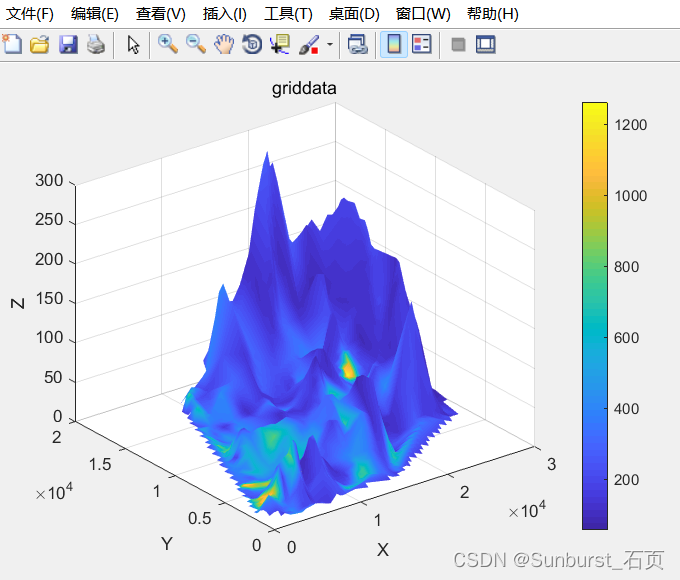展开全文矩阵 图像处理
• 一、一元函数插值已知函数y=f(x)在区间[a,b]上的n+1个不同点的函数值为，若存在一个简单函数F(x), 使，称F(x)为f(x)在区间[a,b]上的插值函数，称(xi, yi)为插值节点。若F(x)为多项式，称为多项式插值(或代数插值) ；...
• MATLAB中的插值函数为interp1，其调用格式为：yi=interp1(x,y,xi,'method')其中x，y为插值点，yi为在被插值点xi处的插值结果；x,y为向量， 'method'表示采用的插值方法，MATLAB提供的插值方法有几种： 'method'是最...
• 实现图像缩放功能的Matlab插值算法研究与比较.pdf
• 关于插值，官网有个小总结，可以直接去参考（从1维到多维），下面是我举的例子。 1, 一维插值interp1(x,y,X1,method) x = linspace(0,10,11) y = sin(x) plot(x,y,'-ro') 插值方法有如下： method=‘nearest’,...
• Matlab实现拉格朗日及牛顿插值法3.用多项式插值法拟合数据实验要求：1.掌握多项式的表示和运算2.拉格朗日插值法的实现(参见吕同富版教材)3.牛顿插值法的实现(参见吕同富版教材)实验内容：1.多项式的表达式和创建；...
• matlab插值实验报告数学实验.doc 新乡学院数学与信息科学系实验报告实验项目名称插值实验所属课程名称数学实验实验类型综合性实验实验日期班级学号姓名成绩一、实验概述【实验目的】掌握用MATLAB插值的方法，了解...
• 插值 x=0:2*pi; y=sin(x); xx=0:0.5:2*pi; %interp1对sin函数进行分段线性插值，调用interp1的时候，默认的是分段线性插值 y1=interp1(x,y,xx); figure plot(x,y,'o',xx,y1,'r') title('分段线性插值') %临近...
• 新乡学院数学与信息科学系 实验报告 实验项目名称 插值实验 所属课程名称 数学实验 实 验 类 型 综合性实验 实 验 日期 班 级 学 号 姓 名 成 绩 一实验概述 实验目的 掌握用Matlab插值的方法了解拉格朗日插值线性...
• 目录 TOC \o "1-3" \h \z \u 一维插值interp1 1 yi = interp1(x,y,xi,method) 1 例1 1 例2 2 二维插值interp2 3 ZI = interp2(X,Y,Z,XI,YI,method) 3 插值方式比较示例 3 例3 3 例4 3 三角测量和分散数据插值 3 数据...
• ## MATLAB插值与拟合

千次阅读 2021-01-16 13:51:40
一.1维插值函数 1.通用接口: 进行1维插值:vq=interp1([x,]v,xq[,method,extrapolation) #参数说明: x,v:分别指定样本点的x,y值;x默认为[1,2...length(v)](v为向量)或[1,2...size(v,1)](v为高维数组) #注意:...
• matlab插值法，试验设计教程，powerpoint
• matlab插值代码解释激烈的 介绍 INT（E）NSE是IN插值的二维（D）-Navier-Stokes-Equations的缩写（双关语）。 这是我在业余时间开发的不稳定的Navier-Stokes解算器。 使用混合的二阶和一阶精确方案对面部速度进行...
• 7种插值算法的c++代码实现，1 拉格朗日插值(POLINT) 2 有理函数插值(RATINT) 3 三次样条插值(SPLINE(二阶导数值)->SPLINT(函数值)) 4 有序表的检索法(LOCATE(二分法), HUNT(关联法)) 5 插值多项式(POLCOE(n2), ...算法
• 四课时matlab插值，通过编程实战掌握具体应用。包括matlab插值一维内插、matlab插值二维内插、matlab插值二维散布点内插、matlab插值三角内插。
• matlab 插值法实验五 插值法5.1实验目的掌握插值的基本思想与方法，会借助数学软件Matlab求解并讨论其收敛性．5.2实验内容1、Lagrange插值法、Newton插值法的Matlab求解方法，在对Runge现象的观察基础上，了解高次......

# matlab插值matlab 订阅